Courses

# SSC CPO Mock Test (Quant Apt) 4

## 50 Questions MCQ Test SSC CPO & Constable - Mock Tests & Previous Year Papers | SSC CPO Mock Test (Quant Apt) 4

Description
This mock test of SSC CPO Mock Test (Quant Apt) 4 for SSC helps you for every SSC entrance exam. This contains 50 Multiple Choice Questions for SSC SSC CPO Mock Test (Quant Apt) 4 (mcq) to study with solutions a complete question bank. The solved questions answers in this SSC CPO Mock Test (Quant Apt) 4 quiz give you a good mix of easy questions and tough questions. SSC students definitely take this SSC CPO Mock Test (Quant Apt) 4 exercise for a better result in the exam. You can find other SSC CPO Mock Test (Quant Apt) 4 extra questions, long questions & short questions for SSC on EduRev as well by searching above.
QUESTION: 1

Solution:
QUESTION: 2

### Ram is 30 yrs younger than his uncle today. Five yrs ago, Ram was $\frac{1}{4}$th as old as his uncle.How old will Ram's uncle be 5 yrs from today?

Solution: Let at present the ages of uncle and Ram are x and y years respectively. Then
x - y = 30 .....(1)
y - 5 = $\frac{1}{4}$ (x - 5)
⇒ 4y - 20 = x - 5
⇒ x - 4y = -15 .....(2)
On solving, we get
y = 15 and x = 45
∴ Age of uncle after 5 years
= 45 + 5 = 50 years
QUESTION: 3

### If α and β are the two roots of the equation 2x2-7x-3=0, then find the value of (α+2)(β+2)

Solution:
QUESTION: 4
The solution of 2x+3y=2 and 3x+2y=2 can be represented by a point in the coordinate planes in
Solution:
QUESTION: 5

If the quality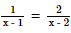is satisfied by x, then the value of x will be

Solution: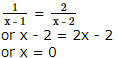QUESTION: 6

A bicycle wheel makes 500 revolutions in moving 1.1 km. The diameter of the wheel is

Solution: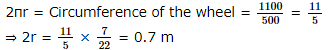QUESTION: 7

Let PQR be a right angled isosceles triangle right angled at P (2, 1). If the eqaution of the line QR is 2x + y = 3, then the equation representing the pair of lines PQ and PR is

Solution:
QUESTION: 8

Diagonals of a rhombus are in the ratio 3:4 and its area is 2400 cm2. Side of the rhombus is

Solution:

Let the diagonals of a rhombus are 3K and 4K. Then
Area of rhombus = 1/2    x product of diagonals
2400 = 1/2 x 3K x 4K
or 400 = K2
or K = 200
∴ The Diagonals are 60 cm and 80 cm
we know that, if a be side of the rhombus and AC and BD be its diagonals then
(AC)2 + (BD)2 = 4 x a2
or (60)2 + (80)2 = 4 x a2
or 3600 + 6400 = 4 x a2
or 10000 = 4a2
or 2500 = a2
or a = 50 cm

QUESTION: 9
A cricketer has a certain average for 10 innings. In the eleventh inning, he scored 108 runs, thereby increasing his average by 6 runs. His new average is:
Solution:
QUESTION: 10

The mean of 30 values was 150. It was detected on rechecking that one value 165 was wrongly copied as 135 for the computation of the mean. Find the correct mean.

Solution: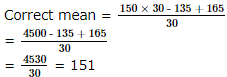QUESTION: 11
Peter invested an amount of Rs. 12,000 at the rate of 10 p.c.p.a. simple interest and another amount at the rate of 20 p.c.p.a. simple interest. The total interest earned at the end of one year on the total amount invested became 14 p.c.p.a. Find the total amount invested.
Solution:
QUESTION: 12

If cube root of 175616 is 56, then the value of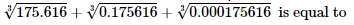Solution:
QUESTION: 13

Chord AB of a circle radius 28 cm subtends an angle of 90º at the centre of the cirlce. The area of the major segment is .... (use π = 3.14)

Solution:

Radius of circle r = 28 cm
π = 3.14
θ = 90º
where θ is the angle subtended at the centre of the circle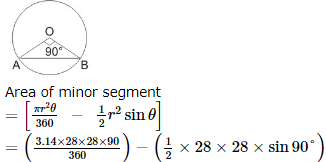= 615.44 - 392
= 223.44
Area of circle = πr2 = 3.14 x 28 x 28 = 2461.76
Area of major segment = Area of circle - Area of minor segment
= 2461.76 - 223.44 = 2238.32 cm2

QUESTION: 14

A publisher supplies books to a bookseller at 20% discount on the list price of Rs. 1574.80. If the book seller sells books at list price and if his expenses for frieght etc. are Rs. 45.40, determine his profit.

Solution: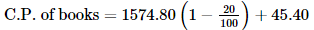= 1259.84 + 45.40 = 1305.24
Profit = 1574.80 - 1305.24 = 269.56

QUESTION: 15

If ABCD is a cyclic quadrilateral in which ∠DAC=27°, ∠DBA=50° and ∠ADB=33°, then ∠CAB is equal to :

Solution:
QUESTION: 16

If P, Q, R and S are the mid-points of sides AB, BC, CD and DA respectively of quadrilateral. ABCD, E, F, G and H are the mid-points of sides PQ, OR, RS and SP of quadrilateral PQRS and area of ∆HGF = 9 sq. units then area of quaqdrilateral ABCD is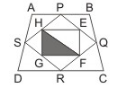Solution: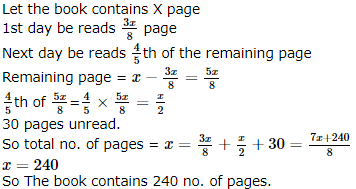QUESTION: 17

A boy read 3/8th of the book on one day and 4/5th of the remainder on another day. If there were 30 pages unread, then how many pages did the book contain?

Solution:QUESTION: 18

Circles, C(O,5 cm) and C(O,3cm) are two concentric circles. PQ is a chord of the larger circle and is a tangent to the smaller circle at R. Then the measure of ∠ORP is

Solution:
QUESTION: 19

Angles of a triangle have measures (x + 40)°, (2x + 20)° and (3x)°. The triangle is

Solution:

(x + 40) + (2x + 20) + (3x) = 180
⇒⇒ x = 20
Hence each angle is 60° or the triangles are equilateral.

QUESTION: 20
The least number ,which when increased by 1,is exactly divisible by 12,18,24,32,40,is
Solution:
QUESTION: 21
The qutab Minar casts a shadow 150 m long at the same time when the Vikas Minar casts a shadow of 120 m long on the ground.If the height of the Vikas Minar is 80 m,find the height of the Qutab Minar.
Solution:
QUESTION: 22
If two-seventh of one-fifth of number is 25, what is 12% of that number?
Solution:
QUESTION: 23
If the sum of two numbers is 3 and the sum of their squares is 12,then their product is equal to
Solution:
QUESTION: 24
16% increase in the train fare resulted in a 6% decrease in the passenger traffic.Still the daily collection of a station increased by Rs 5,424. Find the daily collection of the station before the increase in the fare.
Solution:
QUESTION: 25
Pipes A and B running together can fill a cistern in 6 minutes. If B takes 5 minutes more than A to fill the cistern, then the times in which A and B will fill the cistern separately will be respectively
Solution:
QUESTION: 26

If the side of a triangle are 13, 14, 15, then the radius of the incircle is

Solution:
QUESTION: 27
A man purchased a box full of pencils at the rate of 7 for Rs.9 and sold all of them at the rate of 8 for Rs.11. In this transaction, he gained Rs.10. How many pencils did the box contain?
Solution:
QUESTION: 28
A house and a shop were sold for Rs 1 lakh each. In this transaction,the house sale resulted into 20% loss whereas the shop sale into 20% profit. The entire transaction resulted in
Solution:
QUESTION: 29
If a:b:c=2:3:4, then 1/a : 1/b : 1/c is equal to
Solution:
QUESTION: 30
Two numbers are in the ratio of 5:7. On diminishing each of them by 40, they become in the ratio 17:27. The difference of the numbers is
Solution:
QUESTION: 31
The sum of the series (1/2) + (1/3) + (1/6) + .... upto 9 terms is
Solution:
QUESTION: 32
Rs 5000 earned Rs 300 interest in 2 yrs. The rate of interest is
Solution:
QUESTION: 33

If √6 = 2.449, then the value of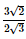is close to

Solution:
QUESTION: 34
X and Y start from the same point and run around a circular stadium, whose circumference is 4200 m, at the rate of 500 m and 700 m per minute respectively in the opposite directions. They will meet each other in
Solution:
QUESTION: 35

Three men, four women and six children can complete a work in seven days. A woman does double the work a man does and a child does half the work a man does. How many women alone can complete this work in 7 days?

Solution:

Let part of work done/day by a man be x.
∴ Part of work done/day by a woman = 2x,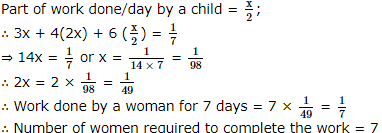QUESTION: 36

If a circular wire of radius 10 cm is cut and spread on the circumference of the circle of diameter 1 m, then the angle subtended by the wire at the centre is :

Solution:

Circumference of a circle of radius 10 cm = 2 πr
= 2 π × 10 = 20 π
diameter of another circle = 1m = 100 cm
radius = 10021002 = 50 cm
subtended angle at the centre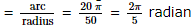QUESTION: 37

If [(2sinα)/(1+cosα+sinα)]=y, then [(1-cosα+sinα)/(1+sinα)]=

Solution:
QUESTION: 38
The greatest value of the function √3 sinx + cosx is
Solution:
QUESTION: 39
A cone of height 9 cm with diameter of its base 18 cm is carved out from a wooden solid sphere of radius 9 cm. The percentage of the wood wasted is :
Solution:
QUESTION: 40

A solid cube of side 20 cm is cut into 8 equal cubes. The total surface area increases by

Solution:

Edge of the cube = 20 cm
Volume of the cube = (Edge)3 cm3
= (20)3 cm3
= 8000 cm3
Number of smaller cube = 8
Volume of the 1 smaller cube = 8000/8 cm3 = 1000cm 3
Side of the smaller cube = a
∴ a3 = 1000
⇒ a = 10 cm
Surface area of the cube = 6(side)2
Surface area of solid cube whose side is 20 cm = 6 x (20)2 = 2400 cm2
Surface area of cube whose side is 10 cm = 6 x (10)2 = 600 cm2
Surface area of 8 small cube = 8 x 600 cm2 = 4800 cm2
∴ Total surface area increase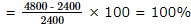QUESTION: 41

The volume of material of a hollow sphere with external radius 10 cm and internal diameter 6 cm is nearly

Solution:
QUESTION: 42

Number of Computers Manufactured and Sold by Various Companies in a year (Number in Lakhs)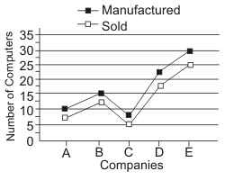Q. What is the respective ratio of the number of computers manufactured by companies A and C together to the number of computers sold by companies A and C together ?

Solution:
QUESTION: 43

Number of Computers Manufactured and Sold by Various Companies in a year (Number in Lakhs)Q. What is the difference between the average number of computers manufactured by all the companies together and the average number of computers sold by all the companies together ?

Solution: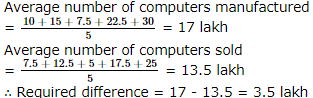QUESTION: 44

Number of Computers Manufactured and Sold by Various Companies in a year (Number in Lakhs)Q. The number of computers by company B are what percent of the number of computers manufactured by company B? (rounded off to two digits after decimal)

Solution: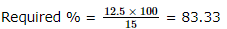QUESTION: 45

Number of Computers Manufactured and Sold by Various Companies in a year (Number in Lakhs)Q. The number of computers manufactured by company D are what percent of the number of computers manufactured by company E ?

Solution: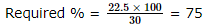QUESTION: 46

The gross investment of Life Insurance Corporation of India (in crore of rupees) in different sectors are shown in the pie chart given below. The letters denoting the various sectors are:
A = Private sector
B = Socially-oriented sector(Plan)
C = Socially-oriented sectors(Non-Plan)
D = Securities guranteed by the central government
E = State government securities
F = Central government securites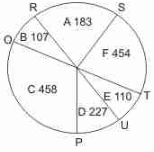Q. The percentage of gross investments in State Government Securities is nearly:

Solution:

Required % = 110/1539 × 100 = 7.1%

QUESTION: 47

The gross investment of Life Insurance Corporation of India (in crore of rupees) in different sectors are shown in the pie chart given below. The letters denoting the various sectors are:
A = Private sector
B = Socially-oriented sector(Plan)
C = Socially-oriented sectors(Non-Plan)
D = Securities guranteed by the central government
E = State government securities
F = Central government securitesQ.  The central angle made by the sector indicating the investments made in the socially oriented sectors is nearly :

Solution: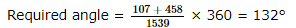QUESTION: 48

The gross investment of Life Insurance Corporation of India (in crore of rupees) in different sectors are shown in the pie chart given below. The letters denoting the various sectors are:
A = Private sector
B = Socially-oriented sector(Plan)
C = Socially-oriented sectors(Non-Plan)
D = Securities guranteed by the central government
E = State government securities
F = Central government securitesQ.  The investment in Private sector is nearly how much percent higher than the investment in the State Government Securities?

Solution:QUESTION: 49

The gross investment of Life Insurance Corporation of India (in crore of rupees) in different sectors are shown in the pie chart given below. The letters denoting the various sectors are:
A = Private sector
B = Socially-oriented sector(Plan)
C = Socially-oriented sectors(Non-Plan)
D = Securities guranteed by the central government
E = State government securities
F = Central government securitesQ.  The ratio , of the area of the circle above ROU to the area of the circle below it is nearly :

Solution: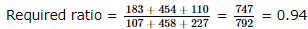QUESTION: 50

The gross investment of Life Insurance Corporation of India (in crore of rupees) in different sectors are shown in the pie chart given below. The letters denoting the various sectors are:
A = Private sector
B = Socially-oriented sector(Plan)
C = Socially-oriented sectors(Non-Plan)
D = Securities guranteed by the central government
E = State government securities
F = Central government securitesQ.  The investments in Socially oriented sectors (plan and non plan) is .... than the investement in the Government Securities (Central and the state ) by ....

Solution:

Investment in socially oriented sector (Plan and Non Plan)
= (107 + 458) = Rs 65 crores
investment in central and State Government Securities
= (454 + 110) = Rs 514 crores
∴ Difference = (565 - 564) = Rs 1 crore